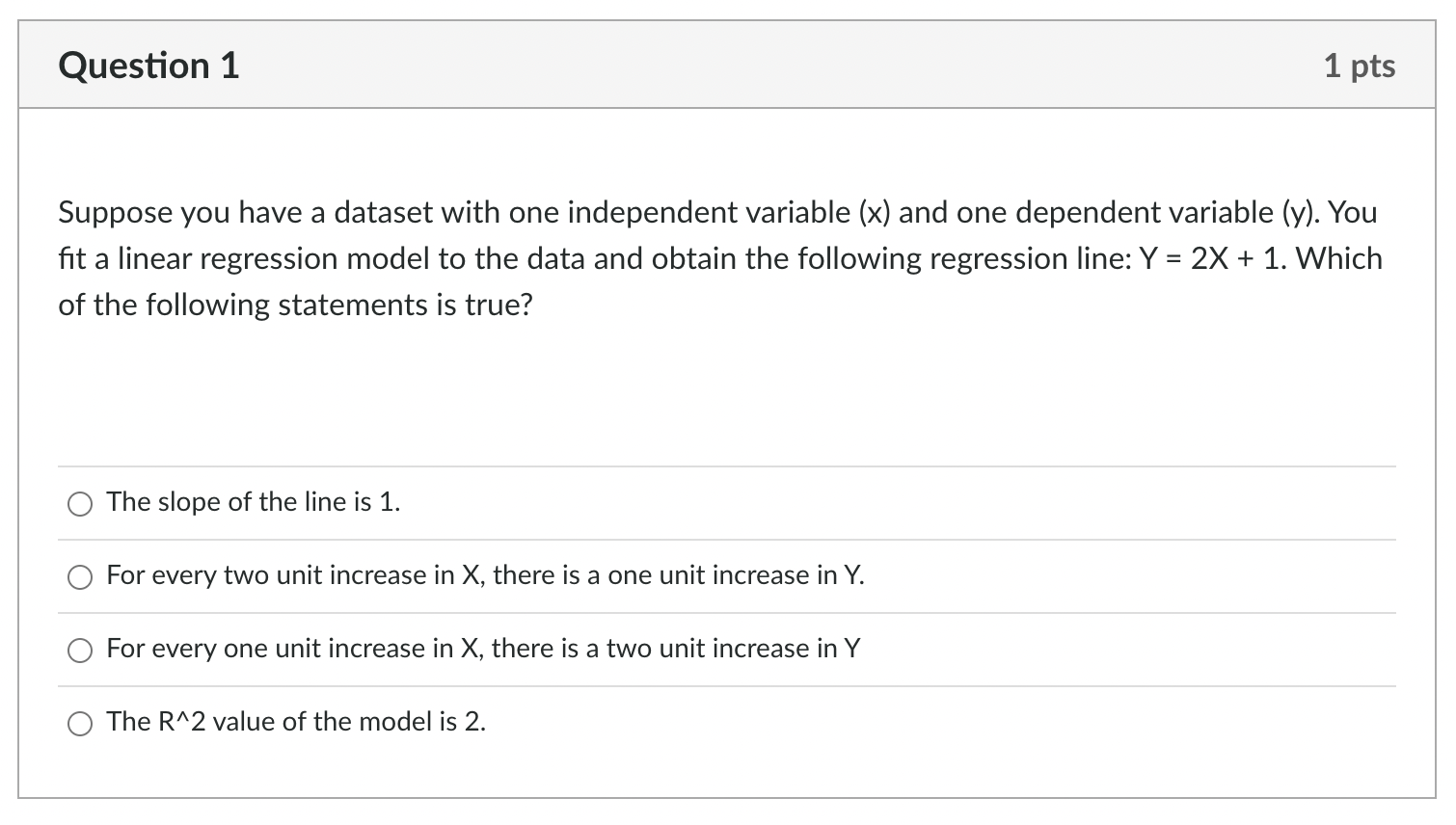Home / Expert Answers / Statistics and Probability / suppose-you-have-a-dataset-with-one-independent-variable-x-and-one-dependent-variable-y-you-pa641

# (Solved): Suppose you have a dataset with one independent variable ( x ) and one dependent variable (y). You ...Suppose you have a dataset with one independent variable ( ) and one dependent variable (y). You fit a linear regression model to the data and obtain the following regression line: . Which of the following statements is true? The slope of the line is 1. For every two unit increase in , there is a one unit increase in . For every one unit increase in , there is a two unit increase in The value of the model is 2 .

We have an Answer from Expert